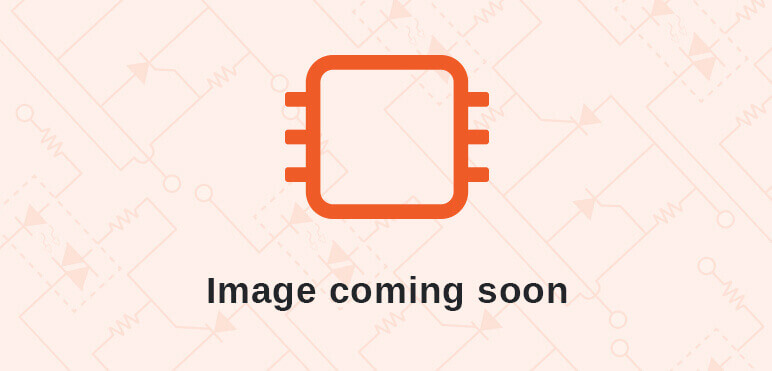# Digital PID Controller - Digital Proportional Integral Derivative Controller Algorithm### Details

Category: DSP Core

Created: July 10, 2012

Updated: January 27, 2020

Language: Verilog

### Other project properties

Development Status: Stable

Additional info: Design done, FPGA proven, Specification done

WishBone compliant: Yes

WishBone version: n/a

## Description

The PID controller IP core performs digital proportional–integral–derivative controller (PID controller) algorithm. The algorithm first calculates the error between a measured value (PV) and its ideal value (SP), then use the error as an argument to calculate the manipulate value(MV). The MV will adjust the process to minimize the error. It can be used to calculate duty cycle for PWM (Pulse Width Modulation).

Features
• 16-bit signed coefficient and data input: Kp, Ki, Kd, SP and PV.
• 32-bit signed u(n) output.
• Containing one high speed 32-bit prefix-2 Han-Carlson adder and one high speed pipelined 16x16-bit multiplier.
• Latency from input of PV to finished calculation and update of u(n) is 9 clock cycles.
• Ki, Kp, Kd, SP, PV can be updated anytime after reset.
• After every update of Kp or Kd, register Kpd which stores Kp+Kd will be calculated and updated.
• After every update of PV, calculation and update of e(n), e(n-1), sigma and u(n) will be triggered in sequence.
• Overflow register records overflow signals when calculating Kpd, e(n), e(n-1), u(n) and sigma.
• Using 2278 of 4608 (49%) Core Cells in Actel A2F200M3F FPGA and running at 100MHz clock frequency.
• Wishbone B4 compliant interface. Support 16-bit, 32-bit and 64-bit bus width.